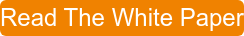Bob Bickler

Recently, one of our readers wrote and asked how to determine solvent strength in normal-phase flash chromatography. This is an excellent question because solvent strength is one of several factors impacting flash chromatography performance.

In this post I will explain how solvent strength can easily be determined.

In previous posts I have discussed the importance of TLC and setting the proper solvent strength in optimizing flash separations.  Each solvent has its own relative strength vs. polar sorbents such as silica and alumina.

When performing TLC and evaluating different solvent combinations it is often beneficial to have solvent blends with similar overall strength, especially as it relates to your targeted compound. So, if you have determined that a few different solvent blends can provide a separation of your mix, performing an “apples-to-apples” comparison at the same solvent strength can be very helpful in determining which blend is optimal for your sample purification.

How do we calculate the strength of a solvent blend? Well, it is simply summing the product of the amount of each solvent and its strength, Equation 1.

Weak solvent portion x weak solvent strength = a

Strong solvent portion x strong solvent strength = b       Equation 1

a + b = total solvent strength

For example, to calculate the strength of a 70:30 mix of hexane and ethyl acetate we find the strength for each solvent (Table 1) and multiply it by the fraction amount of that solvent. Then add the result for each solvent’s calculation to determine the blend’s strength.

Table 1. Solvent strengths of pure solvents relative to silica

 Solvent Strength Selectivity Hexane 0.01 0 Heptane 0.01 0 Cyclohexane 0.04 0 Toluene 0.24 VII Dichloromethane 0.32 V Ethyl ether 0.40 I Ethyl acetate 0.43 VI Acetone 0.50 VI Acetonitrile 0.51 VI Tetrahydrofuran 0.53 III Isopropanol 0.60 II Ethanol 0.65 II Methanol 0.71 II Water 1.00 VIII

Ethyl acetate has a strength of 0.43 and hexane has a strength of 0.01, so the calculation for strength of the 70:30 mix becomes…

Hexane                0.7 x 0.01 = 0.007

Ethyl acetate      0.3 x 0.43 = 0.129

Total strength                         0.136

In another post I show an example where the application of equal solvent strength was used to help with the purification of a mix containing three compounds, Figure 1.

In this case solvent systems of DCM/methanol (9:1) and DCM/acetonitrile (8:2) were used.  The acetonitrile content was adjusted so that it had the same strength as the DCM/methanol solution (0.36).  With both solvent systems the targeted compound had the same approximate Rf value (0.34) by TLC.  However, because the solvent selectivity is different between methanol and acetonitrile, we were able to resolve all three compounds using DCM/MeCN.Figure 1. DCM-MeOH separation (top) and DCM-MeCN separation at equal solvent strength, 0.34 separate the target molecule (last peak) from the other compounds. The use of acetonitrile, however, improves the separation vs. methanol.

How were the solvent strengths determined?  By using the formula above. See Table 2 for the calculations.

Table 2. Solvent strength determinations for DCM/MeOH and DCM/MeCN blends

 Solvent Amount Strength Total DCM 0.9 0.32 0.288 MeOH 0.1 0.71 0.071 Totals 1 0.359 Solvent Amount Strength Total DCM 0.8 0.32 0.256 MeCN 0.2 0.51 0.102 Totals 1 0.358

If you want to learn about our latest in flash chromatography, download the white paper "Successful Flash Chromatography":